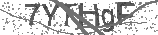# CLEVER MACHİNE

237 Views

Formula to Calculate How Many Watts Electrical Appliances Consume

It is quite easy to calculate the amount of electricity consumed by a device in kilowatt-hours, or kWh! To calculate the electricity consumption, it will be sufficient to consider the following three factors.

Capacity of the device whose consumption we want to calculate in watts

Daily usage time of the device whose electricity consumption will be calculated

The number of days in a year that the device whose electricity consumption is to be calculated is used

How Much Electricity Do Household Appliances Consume?

The point to be underlined is this. The calculation to be made will provide an approximate value. Although the maximum wattage operating ranges are written on the labels of the devices, it should not be overlooked that it can consume less.

We can determine the maximum watt consumption of the device by looking at the labels on it. You can see examples below.

How Do We Know How Many Watts Electrical Appliances Consume From Product Labels?

According to the label, the device below operates in the range of 1850 to 2000 watts.

Home Appliances Electricity Consumption Tags

This device uses 1100 to 1200 watts of electricity. If we were to make calculations, the healthiest thing to do is to take the maximum value, i.e. 1200 watts, as a basis.

How Much Electricity Do Household Appliances Consume? Ticket

The device in our last example works with 275 Watts of power. Let's calculate the electricity consumption of this device together.

How Much Electricity Do Household Appliances Consume? Calculation from label

The device power is calculated as 275W, that is, 275W/1000=0.275kW. Therefore, 1 hour electricity consumption will be 0.275 kW x 3 hours, that is, 0.825 kW. If we multiply the 0.825 kW consumption by 30 days, we find that this device consumes 24.75 kW of electricity per month. Only one transaction remains. Find out how much this device charges the invoice, including taxes.

If you multiply the consumption value with the current electricity unit price, you can calculate your daily electricity cost. For current electricity unit prices, you can take a look at our blog post titled 1kW how much TL. You can visit the EMRA website for current electricity prices.

Category
Forestry & AnimalsBe the first to comment